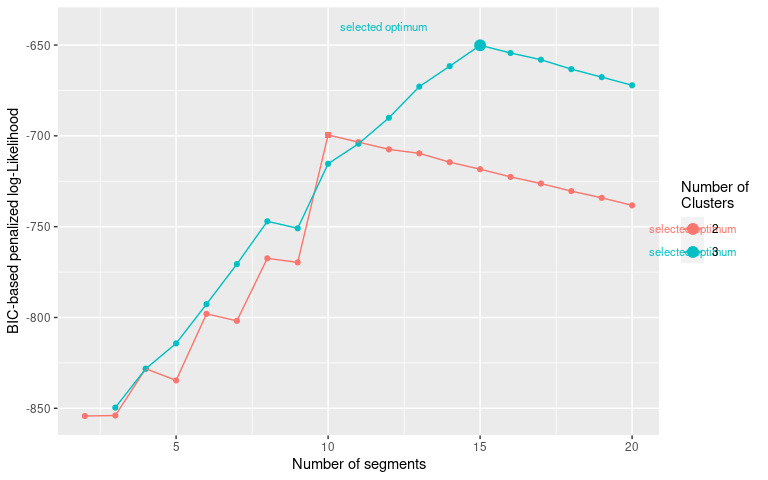# 3 - Exploring Outputs from segclust2d

#### 2021-10-11

library(segclust2d)

# Possible outputs and general functioning

Both segmentation() and segclust() return objects of segmentation-class for which several functions are available (see below).

## General functioning

There are two types of function: (1) some are general and show likelihood for all the different segmentations; (2) other are specific to a given segmentation and requires selecting a number of segments and of clusters (if applicable).

### Default values for nseg and ncluster

For the functions specific to a given segmentation, if you do not provide as argument the number of segments and of clusters, the functions will automatically select the best arguments based on a penalized log-likelihood as following:

• for outputs of segmentation() the optimal number of segments is selected with Lavielle’s criterium. Other numbers of segments may be provided with arguments nseg.

• for outputs of segclust() the optimal numbers of clusters and segments are selected with a BIC-based penalized criterium. Other parameters may be provided with arguments nseg and ncluster. It is recommended to manually choose the number of clusters based on biological knowledge or careful exploration of the BIC-based penalized likelihood. Once the number of clusters was chosen (either manually or automatically) it is recommended to select the number of segments using the automatic BIC-based penalized likelihood criterium.

### Graphical outputs

All plot methods use ggplot2 package and return ggplot objects that can be further modified and customized using classical ggplot2 (see ggplot2 function reference).

### Default value for order

If you provide argument order = TRUE to a function specific to a segmentation, then the different segments or clusters will be numbered ordered by the variable provided as order.var in the segmentation() or segclust() call.

## List of functions

1. Graphical outputs

For a specific segmentation:

• plot.segmentation to show the segmented time-series, and clusters if applicable.
• segmap to show the results of the segmentation as a labelled path (if applicable).
• stateplot plot summary statistics for all segments or clusters.

Summary for all segmentations:

• plot_likelihood for segmentation() show the log-likelihood of the segmentation for all numbers of segments.
• plot_BIC for segclust() show the BIC-based penalized log-likelihood of the segmentation.clustering for all numbers of segments and clusters.
1. Extracting results

For a specific segmentation:

• augment returns a data.frame with the original data as well as the segment or cluster associated for each data point
• segment returns a data.frame with the beginning and end of each segment
• states for segclust provides a data.frame with summary statistics for all clusters

Summary for all segmentations:

• logLik for segmentation() returns a data.frame with the log-likelihood for all numbers of segments.
• BIC for segclust() returns a data.frame with the BIC-based penalized log-likelihood for all numbers of clusters and segments.

# Examples

As functions for segmentation and segmentation/clustering are very similar, we will show examples mostly for the segmentation/clustering outputs, but the use is very similar, argument ncluster just need to be omitted for obtaining outputs for segmentation.

data(simulmode)
simulmode$abs_spatial_angle <- abs(simulmode$spatial_angle)
simulmode <- simulmode[!is.na(simulmode\$abs_spatial_angle), ]
mode_segclust <- segclust(simulmode,
Kmax = 20, lmin=10, ncluster = c(2,3),
seg.var = c("speed","abs_spatial_angle"),
scale.variable = TRUE)

## plot.segmentation for segmented time-series

plot(mode_segclust, ncluster = 3)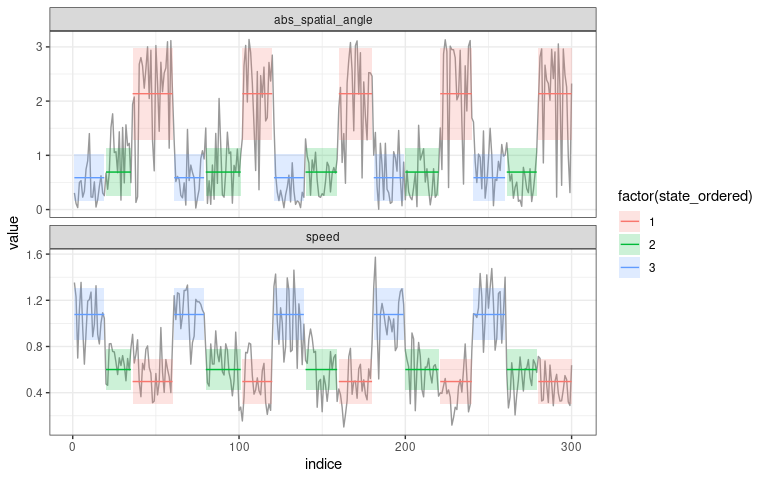## segmap - map the segmentation

segmap() plots the results of the segmentation as a labelled path. This can be done only if data have a geographic meaning. Coordinate names are by default “x” and “y” but they can be provided through argument coord.names.

segmap(mode_segclust, ncluster = 3)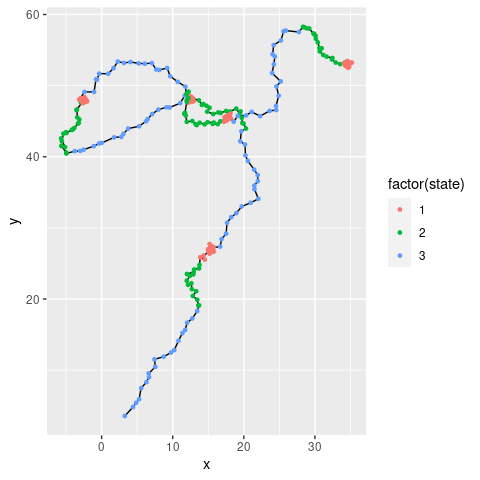## stateplot - plot states statistics

stateplot() shows statistics for each state or segment.

stateplot(mode_segclust, ncluster = 3)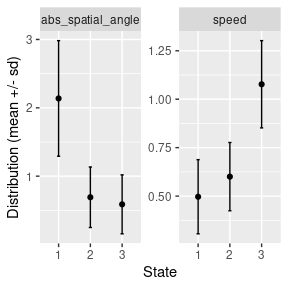## Extract information from segmentation

### augment - get data.frame with segment/cluster information for all points

augment.segmentation() is a method for broom::augment. It returns an augmented data.frame with outputs of the model - here, the attribution to segment or cluster.

augment(mode_segclust, ncluster = 3)

### segment - Extract each segment (begin, end, statistics)

segment() makes it possible to retrieve information on the different segments for a given segmentation. Each segment is associated with the mean and standard deviation for each variable, the state (equivalent to the segment number for segmentation) and the state ordered given a variable - by default the first variable given by seg.var. One can specify the variable for ordering states through the order.var of segmentation() and segclust().

segment(mode_segclust, ncluster = 3)

### states - statistics about each states.

states() returns information on the different states of the segmentation. For segmentation() it is quite similar to segment(). For segclust, however it gives the different clusters found and the statistics associated.

states(mode_segclust, ncluster = 3)

## Get likelihood for all segmentation or segmentation/clustering

### log-Likelihood (segmentation)

logLik.segmentation() return information on the log-likelihood of the different segmentations possible. It returns a data.frame with the number of segments and the log-likelihood.

data("simulshift")
shift_seg <- segmentation(simulshift,
seg.var = c("x","y"),
lmin = 240, Kmax = 25,
subsample_by = 60)
logLik(shift_seg)

plot_likelihood() plots the log-likelihood of the segmentation for all the tested numbers of segments and clusters.

plot_likelihood(shift_seg)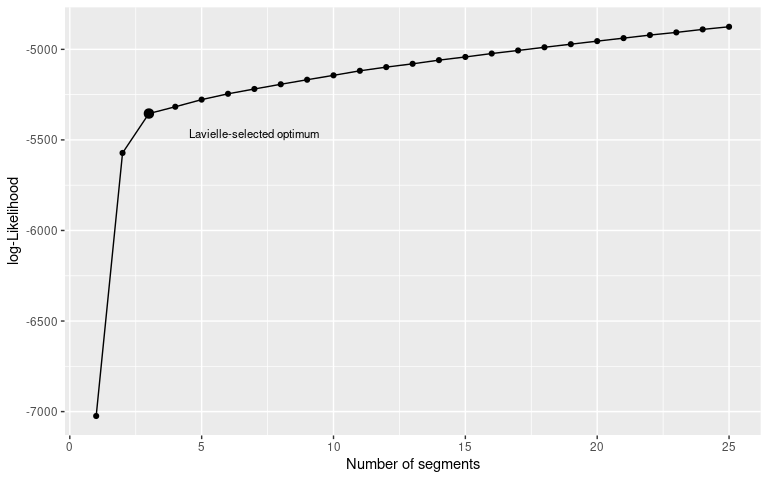### BIC-based penalized likelihood (segclust)

BIC.segmentation() returns information on the BIC-based penalized log-likelihood of the different segmentations possible. It returns a data.frame with the number of segments, the BIC-based penalized log-likelihood and the number of cluster. For segclust() only. Note that this does not truly return a BIC. Here highest values are favored (in opposition to BIC)

BIC(mode_segclust)

plot_BIC() plots the BIC-based penalized log-likelihood of the segmentation for all the tested numbers of segments and clusters.

plot_BIC(mode_segclust)